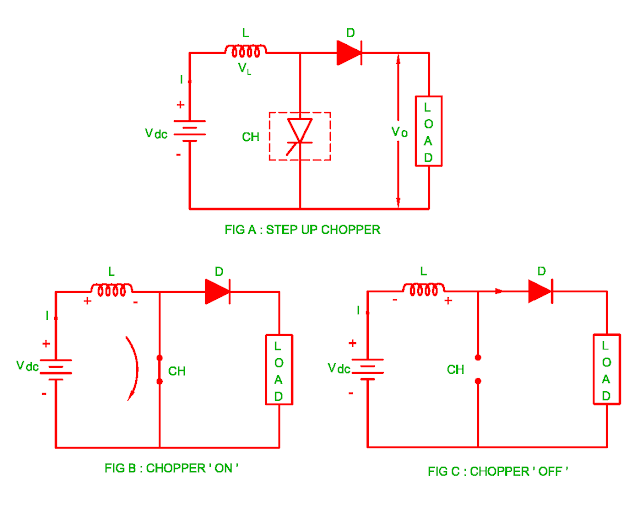## 14/03/2017

### Step up Chopper

• The step up chopper is one in which output DC voltage is greater than the input DC voltage.
• The basic diagram for the step up chopper is shown in the figure A.
•  When the chopper is switched ON during TON time, the energy stored in the inductor via path Vdc – L – CH – Vdc
• The direction of current passing through inductor is shown in the figure B when the chopper CH is switched on.
• When chopper is switched OFF during TOFF time, the current passing through inductor is zero and voltage across inductor is L ( di/dt ).
• The stored energy of inductor is transferred to the load.
• The circuit diagram of step up chopper during chopper OFF time is shown in the figure C.
• The load / output voltage is equal to
VO = Vdc + VL
= Vdc + L (di/dt)
• When chopper is switch ON, the energy stored in the inductor is
Wi = Vdc I TON.......................(1)
• When chopper is switched OFF, the energy stored in the inductor is transferred to the load.
Wo = ( Vo – Vdc )I TOFF.........(2)
• If there are no losses in the system, the input energy is equal to output energy
Vdc I TON = ( Vo – Vdc )I TOFF
Vdc TON = Vo TOFF  – Vdc TOFF
Vdc ( TON + TOFF ) = Vo TOFF
Vo  = [ ( TON + TOFF ) / TOFF ] Vdc
Vo  = [ T / TOFF ] Vdc
OR
Vo  = [ T / ( T –TON ) ] Vdc
Vo  = [ 1 / ( 1 – TON / T ) ] Vdc
Vo  = [ 1 / ( 1 – K ) ] Vdc
When K = 0 ( chopper is in OFF condition )
Vo  =  Vdc
When K = 1 ( chopper is in OFF condition )
Vo  =
• When the duty cycle lies is in the range of 0 < K < 1, the output voltage lies is in the range of Vdc < Vo < ∞.

### Application of DC Step up Chopper

• The application of step up chopper is in the regenerative braking of DC Motor
• The output voltage is greater than the input voltage therefore the DC Motor works as DC generator and load current flows from load to supply side.